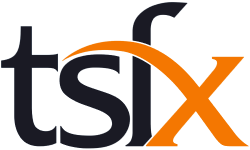# Specialist Mathematics Course Catalogue

Mathematics is the study of function and pattern in number, logic, space and structure, and of randomness, chance, variability and uncertainty in data and events. It is both a framework for thinking and a means of symbolic communication that is powerful, logical, concise and precise. Mathematics also provides a means by which people can understand and manage human and natural aspects of the world and inter-relationships between these. Essential mathematical activities include: conjecturing, hypothesising and problem posing; estimating, calculating and computing; abstracting, proving, refuting and inferring; applying, investigating, modelling and problem solving.

Specialist Mathematics Units 1 and 2 comprise a combination of prescribed and selected non-calculus based
topics and provide courses of study for students interested in advanced study of mathematics, with a focus on
mathematical structure and reasoning. They incorporate topics that, in conjunction with Mathematical Methods
Units 1 and 2, provide preparation for Specialist Mathematics Units 3 and 4 and cover assumed knowledge and
skills for those units

Specialist Mathematics Units 3 and 4
are designed to be taken in conjunction with Mathematical Methods
Units 3 and 4, or following previous completion of Mathematical Methods Units 3 and 4. The areas of study extend
content from Mathematical Methods Units 3 and 4 to include rational and other quotient functions as well as other
advanced mathematics topics such as complex numbers, vectors, differential equations, mechanics and statistical
inference. Study of Specialist Mathematics Units 3 and 4 assumes concurrent study or previous completion of
Mathematical Methods Units 3 and 4.

## Course List

To filter the course list, enter your search text into the below field

For example, entering Unit 3 into the search field would show all Unit 3 Specialist Mathematics Courses.
\Learnworlds\Codeneurons\Pages\ZoneRenderers\CourseCards